﻿ 基于改进遗传算法的电力系统保护CPS关键业务路由优化

# 基于改进遗传算法的电力系统保护CPS关键业务路由优化A CPS Key Service Routing Optimization Approach of Power System Protection Based on Improved Genetic Algorithm

Abstract: CPS (Cyber-Physical System) of power system is an important feature of smart grid. At present, there is relatively little research on the spatial impact of the system protection information space on the power system in the CPS. Therefore, it is necessary to focus on research to solve the problems of safe, stable and reliable operation in power. This paper proposes an improved genetic algorithm to solve the optimization problem of the network service routing path after the communication link is interrupted. This method first analyzes the impact of cross-space risk propagation, and then analyzes the influence of net-work communication link interruptions on the power grid through impacts such as power flow impact, average service latency, and the whole network services balance, and finally through an example simulation experiment. It shows that the proposed solution can improve the reliability of the network by ensuring that the optimized path is 49% better than the original path in the service metrics of the entire network, based on the effect of delay and the impact of grid power flow.

1. 引言

2. 系统保护通信网通信链路中断对电力CPS的影响分析

2.1. 跨空间风险传播影响风险Figure 1. Interaction between information space and power space

$\left\{\begin{array}{l}{A}_{ij}\ne 0\text{}节点\text{\hspace{0.17em}}i\text{\hspace{0.17em}}到节点\text{\hspace{0.17em}}j\text{\hspace{0.17em}}的时延为{A}_{ij}\\ {A}_{ij}=0\text{}节点\text{\hspace{0.17em}}i\text{\hspace{0.17em}}到节点\text{\hspace{0.17em}}j\text{\hspace{0.17em}}的路径不存在\end{array}$

2.2. 对电网潮流的影响

$LOLP=\underset{i\in S}{\sum }{p}_{i}$ (1)

2.3. 对网络业务的影响

2.3.1. 业务平均时延代价

$\stackrel{¯}{T}=\underset{i=1}{\overset{L}{\sum }}{T}_{i}/L$ (2)

2.3.2. 业务均衡度代价

$\stackrel{¯}{L}=\underset{i=1}{\overset{m}{\sum }}{L}_{i}/m$ (3)

$BD=\sqrt{\underset{i=1}{\overset{m}{\sum }}{\left({L}_{i}-\stackrel{¯}{L}\right)}^{2}/m}$ (4)

3. 基于改进遗传算法的系统保护业务路由优化策略

3.1. 数学模型

$R=K\cdot P\cdot I$ (5)

$F=\mathrm{min}R=K\cdot P\cdot \mathrm{min}\left(\alpha {I}_{1}+\beta {I}_{2}+\delta {I}_{3}\right)$

$s.t.\text{\hspace{0.17em}}\left\{\begin{array}{l}{T}_{i}<{T}_{\mathrm{max}}\\ {L}_{i}<{L}_{\mathrm{max}}\\ |{d}_{i,j}|<{P}_{i,j}^{\mathrm{max}}\end{array}$ (6)

3.2. 改进遗传算法

3.2.1. 编码机制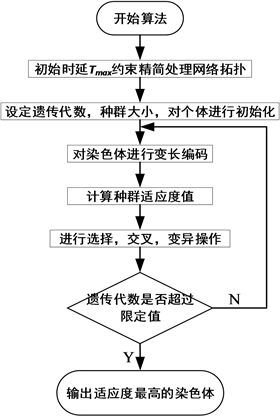Figure 2. The steps of improved genetic algorithm

3.2.2. 初始化种群

3.2.3. 适应度函数

$fitness=\frac{1}{F}=\frac{1}{\alpha {I}_{1}+\beta {I}_{2}+\delta {I}_{3}}$ (7)

3.2.4. 选择操作

3.2.5. 交叉操作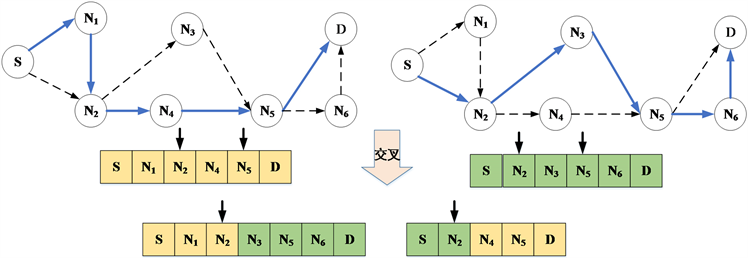Figure 3. A schematic diagram of the crossover operation

3.2.6. 变异操作

4. 仿真验证分析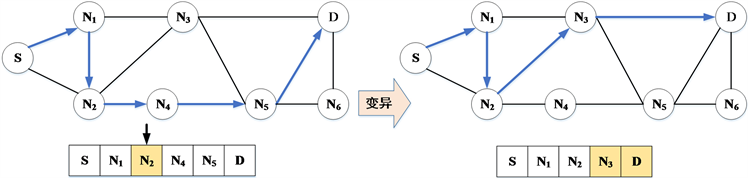Figure 4. A schematic diagram of the mutation operation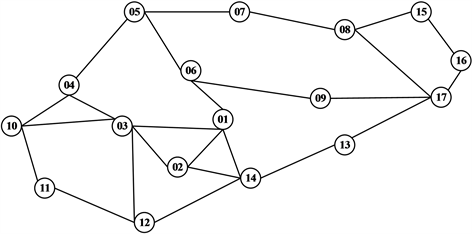Figure 5. The power communication network topologyTable 1. Calculation results of load loss probability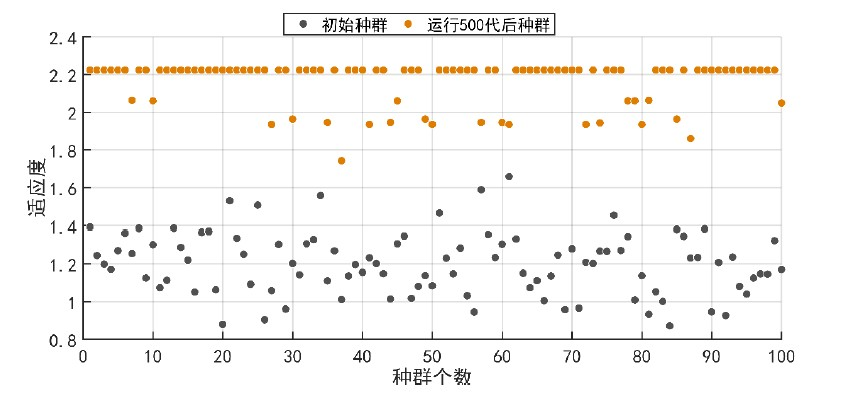Figure 6. Space distribution of initial population and 500 generations after operation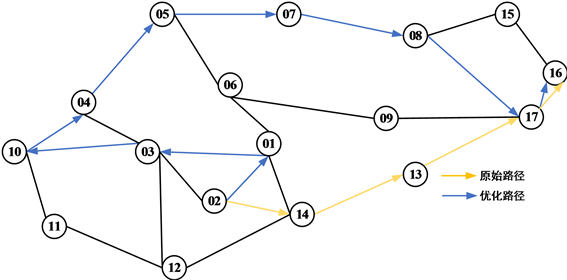Figure 7. Route of service routingTable 2. Comparison of original service paths and optimized service paths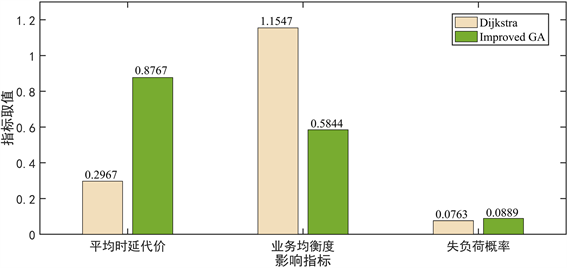Figure 8. Comparative analysis of indicators

5. 结论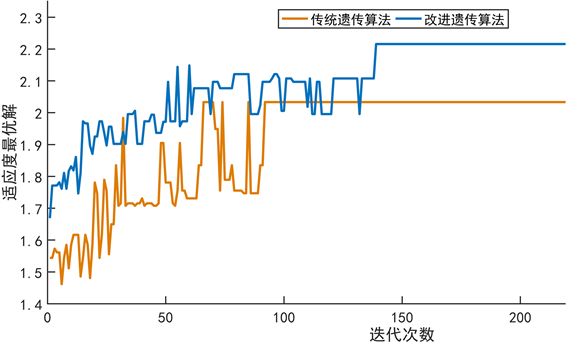Figure 9. The optimal solution produced by each iteration

2017年国家电网公司总部科技项目：系统保护通信网络技术体制及关键技术研究(Grant No. SGXT0000ASJS1700054)。

 郭庆来, 辛蜀骏, 孙宏斌, 等. 电力系统信息物理融合建模与综合安全评估: 驱动力与研究构想[J]. 中国电机工程学报, 2016, 36(6): 1481-1489.

 王智慧, 汪洋, 秦璇, 李艳, 波王湖. 系统保护业务需求分析及通信技术研究[J]. 电力建设, 2017, 38(5): 116-123.

 Zhu, W.T. and Jovica, V.M. (2017) Cyber-Physical System Failure Analysis Based on Complex Network Theory. IEEE EUROCON 2017-17th International Conference on Smart Technologies, Ohrid, 571-575.

 罗泳, 李永丽, 李仲青, 等. 考虑隐性故障的继电保护系统可靠性分析及评估[J]. 电力系统保护与控制, 2014, 42(1): 84-89.

 郭庆来, 辛蜀骏, 王剑辉, 等. 由乌克兰停电事件看信息能源系统综合安全评估[J]. 电力系统自动化, 2016, 40(5): 145-147.

 俞斌, 郭创新, 王越, 等. 考虑信息系统作用的电力系统可靠性研究[J]. 电力系统保护与控制, 2013, 41(7): 7-13.

 范文礼, 刘志刚. 隐性故障对小世界电网连锁故障的影响分析[J]. 电力系统自动化, 2013, 37(21): 23-28.

 汤奕, 韩啸, 吴英俊, 等. 考虑通信系统影响的电力系统综合脆弱性评估[J]. 中国电机工程学报, 2015, 35(23): 6066-6074.

 叶夏明, 文福栓, 尚金成, 等. 电力系统中信息物理安全风险传播机制[J]. 电网技术, 2015, 39(11): 3072-3078.

 刘洋, 马进, 张籍, 等. 考虑继电保护系统的新一代智能变电站可靠性评估[J]. 电力系统保护与控制, 2017, 45(8): 147-154.

 韩小涛, 尹项根, 张哲. 故障树分析法在变电站通信系统可靠性分析中的应用[J]. 电网技术, 2004, 28(1): 56-59.

 曾瑛, 朱文红, 邓博仁, 等. 基于电网影响因子的电力通信网关键节点识别[J]. 电力系统保护与控制, 2016, 44(2): 102-108.

 谷松林. 基于广域保护系统的距离后备保护整定方案[J]. 电力系统保护与控制, 2016, 44(1): 40-47.

 张天宇, 罗凤章, 王成山, 等. 信息系统对微电网运行可靠性的影响分析[J]. 电力系统自动化, 2016, 40(23): 28-35.

 彭静, 卢继平, 汪洋, 等. 广域测量系统通信主干网的风险评估[J]. 中国电机工程学报, 2010(4): 84-90.

 Zhao, P.Y., Yu, P., Ji, C.C., et al. (2016) A Routing Optimization Method Based on Risk Prediction for Communication Services in Smart Grid. International Conference on Network and Service Management (CNSM), Montreal, 377-382.

Top